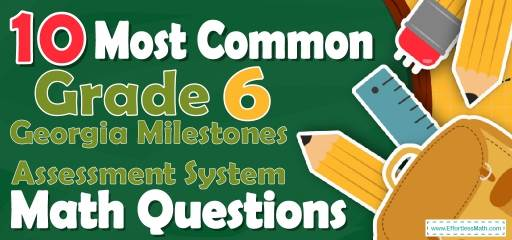# 10 Most Common 6th Grade Georgia Milestones Assessment System Math QuestionsTaking the 6th Grade Georgia Milestones Assessment System sample test questions can make the test takers more and better acquainted with the test conditions and test resources. It is obvious that the readiness of the test takers will lead them to success and it can produce more fruitful results for them. So, in this article, we provide 10 of the 6th Grade Georgia Milestones Assessment System common questions and their step-by-step solutions for your student to practice more.

Make sure to follow some of the related links at the bottom of this post to get a better idea of what kind of mathematics questions your students need to practice.

## 10 Sample 6th Grade Georgia Milestones Assessment System Math Practice Questions

1- What is the equation of a line that passes through points $$(0, 4)$$ and $$(2, 8)$$?

A. $$y = x$$

B. $$y = x + 4$$

C. $$y = 2x + 4$$

D. $$y = 2x – 4$$

2- What is the volume of a box with the following dimensions?

Height $$= 6$$ cm , Width $$= 7$$ cm, Length $$= 9$$ cm

A. $$63 \space cm^3$$

B. $$126\space cm^3$$

C. $$189\space cm^3$$

D. $$378\space cm^3$$

3- Anita’s trick–or–treat bag contains 14 pieces of chocolate, 15 suckers, 16 pieces of gum, 20 pieces of licorice. If she randomly pulls a piece of candy from her bag, what is the probability of her pulling out a piece of sucker?

A. $$\frac{1}{13}$$

B. $$\frac{3}{13}$$

C. $$\frac{14}{65}$$

D. $$\frac{16}{65}$$

4-

In the following rectangle, which statement is false?

A. AD is parallel to BC

B. The measure of the sum of all the angles equals $$360^\circ$$.

C. Length of AB equal to length DC.

D. AB is perpendicular to AC.

5- The area of a rectangular yard is 90 square meters. What is its width if its length is 15 meters?

A. 10 meters

B. 8 meters

C. 6 meters

D. 4 meters

6- Which statement about 4 multiplied by $$\frac{3}{5}$$ must be true?

A. The product is between 1 and 2

B. The product is greater than 3

C. The product is equal to $$\frac{75}{31}$$

D. The product is between 2 and 2.5

7- Which of the following lists shows the fractions in order from least to greatest?
$$\frac{3}{4}, \frac{2}{7}, \frac{3}{8} , \frac{5}{11}$$

A. $$\frac{3}{8}, \frac{2}{7}, \frac{3}{4}, \frac{5}{11}$$

B. $$\frac{2}{7}, \frac{5}{11}, \frac{3}{8}, \frac{3}{4}$$

C. $$\frac{2}{7}, \frac{3}{8}, \frac{5}{11}, \frac{3}{4}$$

D. $$\frac{3}{8}, \frac{2}{7}, \frac{5}{11}, \frac{3}{4}$$

8- A car costing $300 is discounted $$10\%$$. Which of the following expressions can be used to find the selling price of the car? A. $$(300)(0.4)$$ B. $$300-(300×0.1)$$ C. $$(300)(0.1)$$ D. $$300-(300×0.9)$$ 9- What is the missing price factor of number 420? $$420=2^2×3^1×…$$ A. $$2^2×3^1×5^1×7^1$$ B. $$2^2×3^1×7^1×9^1$$ C. $$1^2×2^3×2^1×3^1$$ D. $$3^2×5^1×7^1×9^1$$ 10- If the area of the following trapezoid is equal to A, which equation represent $$x$$? A. $$x = \frac{13}{A}$$ B. $$x = \frac{A}{13}$$ C. $$x=A+13$$ D. $$x=A-13$$ ## Best 6th Grade Georgia Milestones Assessment System Math Practice Resource for 2022 ## Answers: 1- C The slope of the line is: $$\frac{y_2-y_1}{x_2-x_1 }= \frac{8 – 4}{2 – 0}=\frac{4}{2}=2$$ The equation of a line can be written as: $$y-y_0=m(x – x_0 )→y-4 = 2(x – 0)→y-4 = 2x→y = 2x + 4$$ 2- D Volume of a $$box = length × width × height = 6 × 7 × 9 = 378$$ 3- B $$Probability = \frac{number \space of \space desired \space outcomes}{number \space of \space total \space outcomes}=\frac{15}{14+15+16+20}= \frac{15}{65}=\frac{3}{13}$$ 4- D In any rectangle, sides are not perpendicular to diagonals. 5- C Let y be the width of the rectangle. Then; $$15×y=90→y=\frac{90}{15}=6$$ 6- D $$4×\frac{3}{5}=\frac{12}{5}=2.4$$ $$2.4>2$$ $$2.4<3$$ $$\frac{75}{31}=2.419≠2.4$$ $$2<2.4<2.5$$ This is the answer! 7- C Let’s compare each fraction: $$\frac{2}{7}< \frac{3}{8}< \frac{5}{11} < \frac{3}{4}$$ Only choice C provides the right order. 8- B To find the discount, multiply the number ($$100\%$$- rate of discount) Therefore; $$300(100\%-10\%)=300(1-0.1)=300-(300×0.1)$$ 9- A $$420=2^2×3^1×5^1×7^1$$ 10- B The area of the trapezoid is: area= $$\frac{(base 1+base 2)}{2}×height= (\frac{10 + 16}{2})x = A$$ $$→13x = A→x = \frac{A}{13}$$ Looking for the best resource to help you succeed on the Grade 6 Georgia Milestones Assessment System Math test? ## The Best Books to Ace 6th Grade Georgia Milestones Assessment System Math Test ## Related to This Article ### More math articles ### What people say about "10 Most Common 6th Grade Georgia Milestones Assessment System Math Questions - Effortless Math: We Help Students Learn to LOVE Mathematics"? No one replied yet. X 30% OFF Limited time only! Save Over 30% SAVE$5

It was $16.99 now it is$11.99